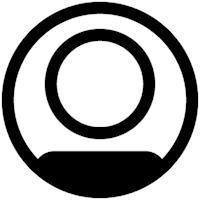# SPSS Statistics

View Only

## Chi-squared test of independence?#### Tom DillonThu November 19, 2020 03:24 PM#### Rick MarcantonioThu November 19, 2020 04:45 PM• #### 1.  Chi-squared test of independence?

Posted Thu November 19, 2020 03:24 PM

Hello,

I am running an experiment on aggression. I have a control and an experimental group. I have administered a 5 point Likert scale to each group. I am interested in their answers, not individual responses, just higher values indicating greater aggression. How can I enter this in SPSS and do the chi-square test of independence based on individuals' overall value within each group?

------------------------------
Tom Dillon
------------------------------

• #### 2.  RE: Chi-squared test of independence?

Posted Thu November 19, 2020 04:45 PM
Edited by Rick Marcantonio Thu November 19, 2020 05:00 PM
Hi, Tom. If I understand correctly, you want to compare the responses for each category of the scale to the control group. SPSS doesn't have a preset way to do that through the menus, but I think this might do what you want.

*I have created some mock data, assuming 10 subjects in each group.

data list free /likert_score group.
begin data.
2 0
3 0
4 0
3 0
4 0
5 0
4 0
4 0
3 0
2 0
5 1
4 1
4 1
3 1
5 1
5 1
5 1
4 1
3 1
5 1
end data.

* Then I aggregate it to get the counts for each possible value of the scale (here I assume the scale goes 1 through 5).

AGGREGATE /OUTFILE=* MODE=replace /BREAK=group
/likert_score_1=CIN(likert_score 1 1)
/likert_score_2=CIN(likert_score 2 2)
/likert_score_3=CIN(likert_score 3 3)
/likert_score_4=CIN(likert_score 4 4)
/likert_score_5=CIN(likert_score 5 5).

* We have to transpose that to get back to columns for the test.

flip variables=likert_score_1 to likert_score_5.

* Rename the variables for clarity.

rename variables (var001 var002=control experimental).

* Now compute chi-square using the control group as the expected counts. If you'd rather,
* you can go into the menu here and do a chi-square specifying equal cell counts for the
* "experimental" variable.

do if (control ne experimental).
compute ev=((experimental-control)**2)/control.
else.
compute ev=0.
end if.

* We need the sum of the expected values...

frequencies variables=ev /format notable /statistics sum.

* Finally, enter the sum as the first argument below.

compute chi_prob=sig.chisq(18.58,4).
execute.

* The value in the last column is the p-value for the chi-square.

------------------------------
Rick Marcantonio
Quality Assurance
IBM
IL
------------------------------

• #### 3.  RE: Chi-squared test of independence?

Posted Thu November 19, 2020 07:30 PM
Wow, that will be helpful in the future so thank you! However, I really just want to look at the overall of the Likert scale. That being the case would I still need to break it into categories, say, 1 strongly disagree 2 disagree, and so forth? Or could I do a variable for scores with the total for each participant and run the chi-square between groups and scores? I apologize if I am not making much sense still in my undergrad. Thanks for any more information or advice you can provide.

------------------------------
Tom Dillon
------------------------------